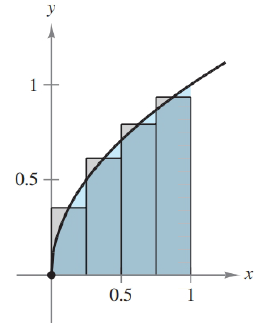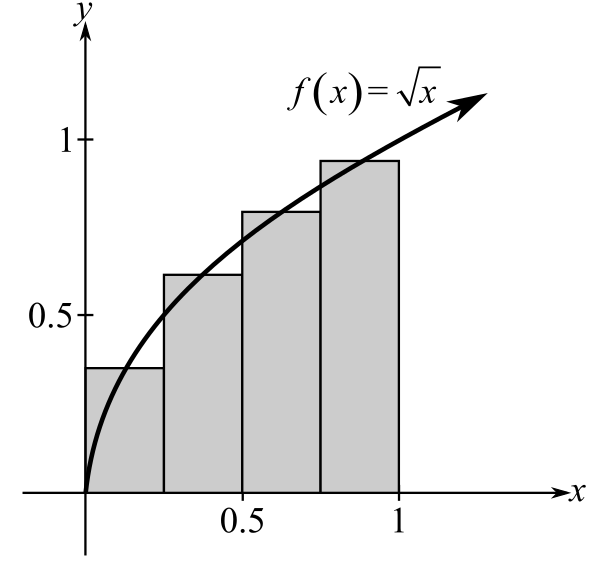Chapter 5.6, Problem 3E### Calculus: An Applied Approach (Min...

10th Edition
Ron Larson
ISBN: 9781305860919

#### Solutions

Chapter
Section### Calculus: An Applied Approach (Min...

10th Edition
Ron Larson
ISBN: 9781305860919
Textbook Problem
1 views

# Approximating the Area of a Plane Region In Exercises 1-6, use the rectangles to approximate the area of the region. Compare your result with the exact area obtained using a definite integral. See Example 1. f ( x ) = x , [ 0 , 1 ]To determine

To calculate: The area bounded by the function f(x)=x between interval [0,1] by mid-point rule. Also compare this approximate area with exact area using definite integral.Explanation

Given Information:

The provided function is f(x)=x. Area of this function is calculated between the interval [0,1] as shown below:

Formula used:

The approximate area of any definite integral baf(x)dx by the use of midpoint rule is calculated by the use of following steps.

Step1: First divide the provided interval of the function into n sub intervals by the use of formula;

Δx=ban where [a,b] are the intervals and n is the subinterval value and Δx is the width.

Step2: Use above subinterval find the midpoint of each sub interval.

Step3: Final step is to obtain approximate area by calculating function f at each mid-point from the use of formula;

abf(x)dxban[f(x1)+f(x2)+f(x3)+....+f(xn)].

Calculation:

Consider the provided function f(x)=x. The graph of provided function is shown below:

In provided graph, interval [0,1] is divided into 4 rectangle.

Now, put n=4, a=0 and b=1 to find the width of each rectangle as;

Δx=104=0.25

Width Δx of each rectangle will be 0.25.

So, the interval [0,1] will be divided into 4 subintervals as;

[0,0.25],[0.25,0.50],[0.50,0.75],[0.75,1.0].

Now, the value of midpoint of each rectangle is calculated as;

[x1,x2]=x1+x22 where x1,x2 are the upper value and lower value of the sub-interval

So, for first rectangle [0,0.25] mid-point is;

0+0.252=0.125

Similarly, for second rectangle [0.25,0.50] mid-point is;

0.25+0.502=0.375

For third rectangle [0.50,0.75] mid-point is;

0.50+0.752=0.625

For last rectangle [0.75,1.0] mid-point is;

0

### Still sussing out bartleby?

Check out a sample textbook solution.

See a sample solution

#### The Solution to Your Study Problems

Bartleby provides explanations to thousands of textbook problems written by our experts, many with advanced degrees!

Get Started

#### Prove formula (b) of Theorem 3.

Single Variable Calculus: Early Transcendentals

#### If f(x) = sin 2x, an upper bound for |f(n + 1)(x)| is 2 2n 2n + 1 22n

Study Guide for Stewart's Single Variable Calculus: Early Transcendentals, 8th

#### Self Check Graph: y=-x.

College Algebra (MindTap Course List)